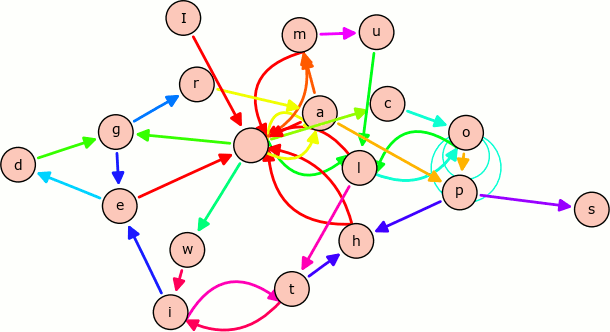# Best Free Linux Computer Algebra Systems

A computer algebra system (CAS) is mathematical software that can manipulate mathematical formulae in a way similar to the traditional manual computations of mathematicians and scientists. This type of system supports a wide range of mathematics including linear algebra, calculus, and algebraic and ordinary differential equations.

A CAS offers a rigorous environment for defining and working with structures such as groups, rings, fields, modules, algebras, schemes, curves, graphs, designs, codes and many others.

They have been extensively used in higher education.

The main features of a CAS include:

• Numerical Computations: The software can determine numerical approximations of solutions, derivatives, integrals, differential equations, etc. Solve, manipulate, and plot functions without needing to generate numeric data. Often problems that cannot be solved explicitly can be solved numerically, and often only a numerical answer is sufficient.
• Data Analysis: Having data is not sufficient; we need to extract useful information from it. There are many algorithms designed for data analysis, most of which involve too much work to be done by manual computations. CAS’s put these algorithms in one place, and offer an environment where the algorithms are easy to implement.
• Data Visualization: CAS’s can graph 2D and 3D functions in a variety of ways. They are also designed to graph vector fields and solutions to differential equations.
• Symbolic Computations: Most of the CAS’s can perform symbolic manipulation of expressions: reducing, expanding, simplifying, derivatives, antiderivatives, etc. Unlike numerical computations, which can exhibit floating-point errors, symbolic computations are determined exactly. They can therefore provide the exact answer to an equation (as opposed to a decimal approximation), and they can express results in terms of a wide variety of previously defined functions.

A CAS automates tedious and sometimes difficult algebraic manipulation tasks. The principal difference between a CAS and a traditional calculator is the ability to deal with equations symbolically rather than numerically.

The chart below offers our rating for each software. Some of the software is very specialized, designed to fill a particular niche. This makes comparisons difficult.To provide an insight into the quality of software that is available, we have compiled a list of 13 impressive algebra systems. There’s general purposes systems as well as specialist software solutions. All of them are open source software.

Let’s explore the 13 algebra systems at hand. For each application we have compiled its own portal page, a full description with an in-depth analysis of its features, screenshots, together with links to relevant resources.

Computer Algebra Systems
SageMathOpen source alternative to Magma, Maple, Mathematica and Matlab
ScilabNumerical computational package
MaximaSystem for the manipulation of symbolic and numerical expressions
OctavePowerful programming language with built-in plotting and visualization tools
SymPyPython library for symbolic mathematics
SINGULARComputer Algebra System for polynomial computations
Macaulay2Software system for research in algebraic geometry
CadabraSymbolic computer algebra system for field theory problems
PARI/GPWidely used algebra system designed for fast computations in number theory
GAPSystem for computational discrete algebra
FriCASFork of Axiom
AxiomGeneral purpose Computer Algebra system
CoCoASystem for doing computations in commutative algebra
 Read our complete collection of recommended free and open source software. The collection covers all categories of software. The software collection forms part of our series of informative articles for Linux enthusiasts. There's tons of in-depth reviews, alternatives to Google, fun things to try, hardware, free programming books and tutorials, and much more.

1.hc speel says:

Can you please share what tool produces the top image, with all the bullets and their relations?

2.student says:

Giac/xcas and qcas (qt front-end) should added to this list

3.student says:

Execellent one: Reduce

This site uses Akismet to reduce spam. Learn how your comment data is processed.